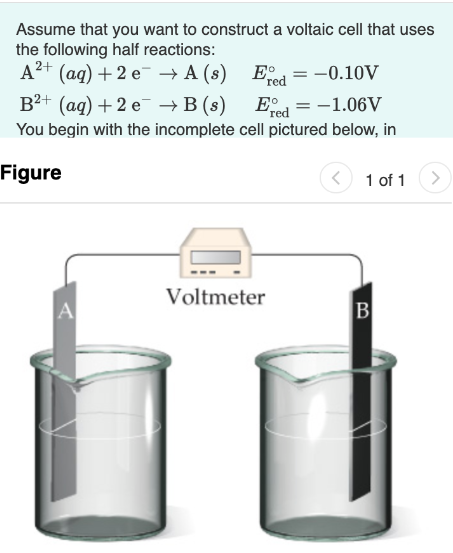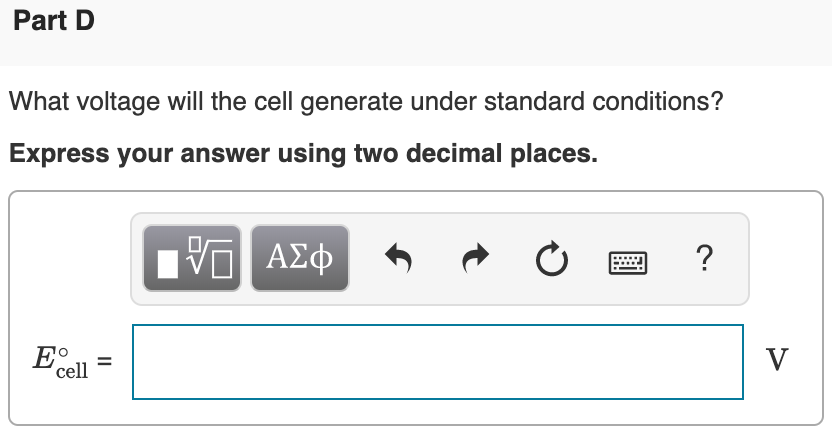# Assume that you want to construct a voltaic cell that uses the following half reactions: A^2+(aq) + 2 e^- → A (s) E°red = -0.10V B^2+(aq) + 2 e^- → B (s) E°red = -1.06V You begin with the incomplete cell pictured below, in What voltage will the cell generate under standard conditions? Express your answer using two decimal places.